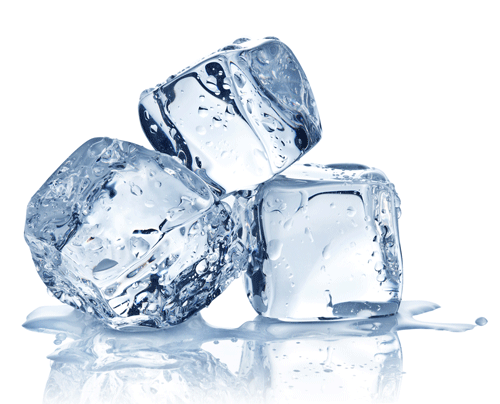10 minutes maximum! Can you do it in 5?

The energy needed to heat up a substance is given by the formula shown here:

ΔQ=m.c.ΔΘ    or    ΔE=m.c.ΔΘ

change in thermal energy = mass x specific heat capacity x temperature change

1. This formula should be used when a substance is...

• A. melting
• B. boiling
• C. heated but staying in the same state.
• D. staying at the same temperature.

2+3. The image shows a large glass vase holding 2 kg of cold water.

Water has a specific heat capacity (c) of 4200 J / Kg 0C.2. The energy needed to heat 2 kg of water by 5 0C is ..

• A. 4200 J
• B. 8400 J
• C. 42000 J
• D. 84000 J

3. If I supply 210 kJ of heat to the same 2 kg of water, how much would the temperature increase?

• A. 25 0C
• B. 50 0C
• C. 100 0C
• D. 250 0C

Q 4-6. These questions are about heating different bars of copper.

Copper has a specific heat capacity of 800 J / Kg 0C.

What are the missing values?Energy Supplied Mass (kg) Temperature Increase (0C) 4. ? 200 J 16 kJ 160 kJ 400 kJ 20 10 5. 8000 J ? 4 10 2 0.5 20 6. 400 kJ 5 ? 4 100 20 200

7. Specific Latent Heat is the energy needed for 1 kg of substance to change state.

Which of these correctly identifies the change of state?

Q=mL   or    E=mL

Energy for change in state = mass x specific latent heat

 Latent Heat of Fusion Latent Heat of Vaporisation A Solid to Liquid Solid to Gas B Liquid to Gas Solid to Gas C Liquid to Gas Solid to Liquid D Solid to Liquid Liquid to Gas

8. These ice cubes have a total mass of 100g. The specific latent heat needed to make them change state is 330 kJ / kg.

What is the energy needed to make them melt from ice at 0 0C to water at 0 0C?Howard wang86 wikimedia commons
• A. 33000 kJ
• B. 3300 kJ
• C. 33 kJ
• D. 3.3 kJ

9. If I supply 1 MJ of energy, roughly how much mass of ice cubes could I melt? (Again all at the melting point - 0 0C).

• A. 30 kg
• B. 3 kg
• C. 0.3 kg
• D. 0.03 kg

10. When the ice cubes are first taken out of the freezer they are really cold. Which graph best shows the change in temperature as the ice cubes eventually melt and continue to warm up?